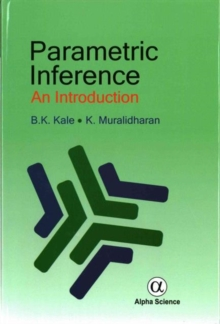Supporting your high street Find out how »
• My Account# Parametric Inference : An Introduction Hardback

#### Description

Parametric Inference: An Introduction discusses the basic concept of sufficient statistic and the classical approach based on minimum variance unbiased estimator.

There is a separate chapter on simultaneous estimation of several parameters.

Large sample theory of estimation, based on consistent asymptotically normal estimators obtained by method of moments, percentile and the method of maximum likelihood is also introduced.

The tests of hypotheses for finite samples with classical Neyman - Pearson theory is developed pointing out its connection with Bayesian approach.

The hypotheses testing and confidence interval techniques are developed leading to likelihood ratio tests, score tests and tests based on maximum likelihood estimators.

#### Information

• Format: Hardback
• Pages: 334 pages
• Publisher: Alpha Science International Ltd
• Publication Date:
• Category: Probability & statistics
• ISBN: 9781842659397

£42.50

£35.25